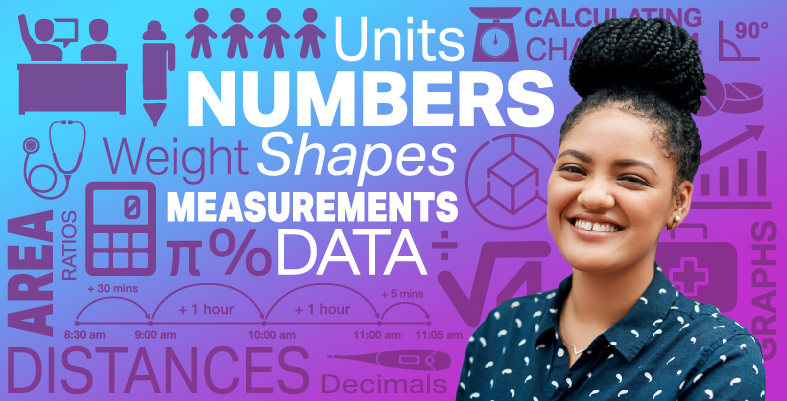Everyday maths for Health and Social Care and Education Support 2

Start this free course now. Just create an account and sign in. Enrol and complete the course for a free statement of participation or digital badge if available.

Free course

# 6.4 Finding the mean from a frequency table

Large groups of data will often be shown as a frequency table, rather than as a long list. This is a much more user friendly way to look at a large set of data. Look at the example below where there is data on how many times, over a month, parents visited a children’s centre.

## Table 16

Number of visits in a monthNumber of parents
16
210
311
416
54
63

We could write this as a list if we wanted to:

• 1, 1, 1, 1, 1, 1, 2, 2, 2, 2, 2, 2, 2, 2, 2, 2, 3, 3, 3, 3, 3, 3, 3, 3, 3, 3, 3 and so on

but it’s much clearer to look at in the table format. But how would you find the mean of this data? Well, there were 6 parents who visited the children’s centre once, that’s a total of 1 × 6 = 6 visits. Then there were 10 parents who visited twice, that’s a total of 2 × 10 = 20 visits. Do this for each row of the table, as shown below.

## Table 17

Number of visits in a monthNumber of parentsTotal visits
161 × 6 = 6
2102 × 10 = 20
3113 × 11 = 33
4164 × 16 = 64
545 × 4 = 20
636 × 3 = 18
Total = 50 parentsTotal = 161 visits

Finally, work out the totals for each column (highlighted in a lighter colour on the table above).

Now you have all the information you need to find the mean; the total number of visits is 161 and the total number of parents is 50 so you do 161 ÷ 50 = 3.22 month per year as the mean average.

Warning! Many people trip up on these because they will find the total visits (161) but rather than divide by the total number of parents (50) they divide by the number of rows in the table (in this example, 6).

If you do 161 ÷ 6 = 26.83, logic tells you, that since the maximum number of visits any parent made was 6, the mean average cannot be 26.83. Always sense check your answer to make sure it is somewhere between the lowest and highest values of the table. For this example, anything below 1 or above 6 must be incorrect!

Have a go at a couple of these yourself so that you feel confident with this skill.

## Activity 11: Finding the mean from frequency tables

1. The table below shows some data about the number of times children were absent from school over a term.

Work out the mean average number of absences.

### Table 18

Number of absencesNumber of children
126
213
30
435
56

1. First, work out the total number of absences by multiplying the absence column by the number of children. Next, work out the totals for each column.

#### Table 19

Number of absencesNumber of childrenTotal number of absences
1261 × 26 = 26
2132 × 13 = 26
303 × 0 = 0
4354 × 35 = 140
565 × 6 = 30
Total = 80Total = 222
• To find the mean do: 222 ÷ 80 = 2.775 mean average.

1. You run a youth club for 16–21-year-olds and are interested in the average age of young people who attend.

You collect the information shown in the table below. Work out the mean age of those who attend. Give your answer rounded to one decimal place.

### Table 20

AgeNumber of young people
163
178
185
1912
206
211

1. Again, first work out the totals for each row and column.

#### Table 21

AgeNumber of young peopleAge × Number
16316 × 3 = 48
17817 × 8 = 136
18518 × 5 = 90
191219 × 12 = 228
20620 × 6 = 120
21121 × 1 = 21
Total = 35Total = 643
• To find the mean you then do:

• 643 ÷ 35 = 18.4 years (rounded to one d.p.)

If you are given all the data in a set and asked to find the mean, it’s a relatively simple process (as long as you have your calculator handy!). What if you are only given some of the data and the mean? How do you go about working out the missing data? The next part of this section deals with these kind of ‘reverse mean’ skills.

FSM_SSH_2